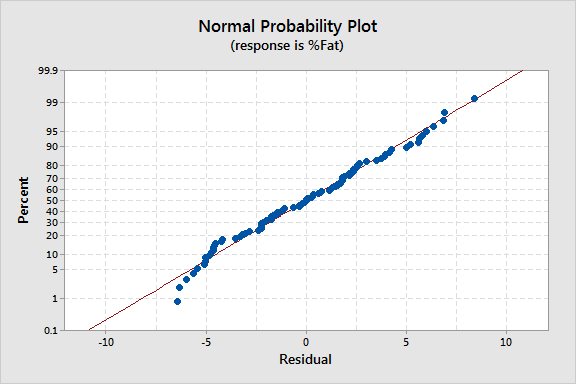#This article was written by Jim Frost. Here we present a summary, with link to the original article.

Ordinary Least Squares (OLS) is the most common estimation method for linear models—and that’s true for a good reason. As long as your model satisfies the OLS assumptions for linear regression, you can rest easy knowing that you’re getting the best possible estimates.

Regression is a powerful analysis that can analyze multiple variables simultaneously to answer complex research questions. However, if you don’t satisfy the OLS assumptions, you might not be able to trust the results.

In this post, I cover the OLS linear regression assumptions, why they’re essential, and help you determine whether your model satisfies the assumptions.## What Does OLS Estimate and What are Good Estimates?

First, a bit of context.

Regression analysis is like other inferential methodologies. Our goal is to draw a random sample from a population and use it to estimate the properties of that population.

In regression analysis, the coefficients in the regression equation are estimates of the actual population parameters. We want these coefficient estimates to be the best possible estimates!

Suppose you request an estimate—say for the cost of a service that you are considering. How would you define a reasonable estimate?

1. The estimates should tend to be right on target. They should not be systematically too high or too low. In other words, they should be unbiased or correct on average.
2. Recognizing that estimates are almost never exactly correct, you want to minimize the discrepancy between the estimated value and actual value. Large differences are bad!

These two properties are exactly what we need for our coefficient estimates!

When your linear regression model satisfies the OLS assumptions, the procedure generates unbiased coefficient estimates that tend to be relatively close to the true population values (minimum variance). In fact, the Gauss-Markov theorem states that OLS produces estimates that are better than estimates from all other linear model estimation methods when the assumptions hold true.

For more information about the implications of this theorem on OLS estimates, read my post: The Gauss-Markov Theorem and BLUE OLS Coefficient Estimates.

## The Seven Classical OLS Assumption

Like many statistical analyses, ordinary least squares (OLS) regression has underlying assumptions. When these classical assumptions for linear regression are true, ordinary least squares produces the best estimates. However, if some of these assumptions are not true, you might need to employ remedial measures or use other estimation methods to improve the results.

Many of these assumptions describe properties of the error term. Unfortunately, the error term is a population value that we’ll never know. Instead, we’ll use the next best thing that is available—the residuals. Residuals are the sample estimate of the error for each observation.

Residuals = Observed value – the fitted value

When it comes to checking OLS assumptions, assessing the residuals is crucial!

There are seven classical OLS assumptions for linear regression. The first six are mandatory to produce the best estimates. While the quality of the estimates does not depend on the seventh assumption, analysts often evaluate it for other important reasons that I’ll cover. Below are these assumptions:

1. The regression model is linear in the coefficients and the error term
2. The error term has a population mean of zero
3. All independent variables are uncorrelated with the error term
4. Observations of the error term are uncorrelated with each other
5. The error term has a constant variance (no heteroscedasticity)
6. No independent variable is a perfect linear function of other explanatory variables
7. The error term is normally distributed (optional)

Why You Should Care About the Classical OLS Assumptions?

## DSC Resources

Views: 15989

Comment

Join Data Science Central